# Fleming's Phase II Procedure

Hypothesis: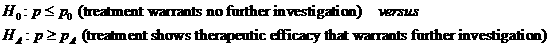Data Input: (Help) (Example)

 Input Results α β p0 pA Nmax Numstage (1 - 3)

Note:

 Variables Descriptions α Probability of type I error β Probability of type II error p True treatment response probability p0 Largest response probability pA Smallest response probability Nmax Maximum number of patients to be recruited Numstage Number of stages in Phase II clinical trial ni Number of patients to be recruited in stage i ai Acceptance points in stage i ri Rejection points in stage i

Help Aids

Application:   Commonly, the central objective of Phase II clinical trials is the assessment of the antitumor ‘therapeutic efficacy’ of a specific treatment regimen. It is of interest to formulate test procedures which can be employed in these trials to decide whether or not this therapeutic efficacy warrants further investigation. One-sample multiple testing procedure is proposed which employs the standard single-stage test procedure at the last test.

Procedure:

1. Enter

a)   value of α, the probability of type I error

b)   value of β, the probability of type II error, or (1-power) of the test

c)   value of p0, the largest response probability

d)   value of pA, the smallest response probability

e)   value of Nmax, the maximum number of patients to be recruited

f)   value of Numstage, the number of stages in Phase II clinical trial.

1. Click the button “Calculate” to obtain

a)    value of ni, the number of patients to be recruited in stage i

b)    value of ai, the acceptance points in stage i

c)   value of ri, the rejection points in stage i.

Assumption:

1.      Assume that a Phase I trial of the experimental treatment regimen has been completed, from which the proper dosages yielding acceptable toxicity have been established.

2.      Probability of type I error is smaller or equal than that of type II error (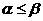).

3.      A maximum of K=1,2 or 3 tests is adopted in the calculator, since more frequent testing would not seem to be necessary in most Phase II trials.

4.      Due to the very limited number of patients who are eligible for a given Phase II study, and also to the large number of treatment regimens generally awaiting Phase II trials, the sample size N for a given trial is frequently fixed in advance.

5.      A certain number of Phase II trials are terminated due to extreme response probability results, not due to other causes such as excessive toxicity or unexpectedly inadequate patient accrual.

Theory:

Typically, a Phase II trial is designed to have a single stage in which N evaluable patients accrue, are treated, and are then observed, so that the number, S, who experience a regression is determined. In a multiple testing procedure patients accrue in several stages, testing being performed at each stage after appropriate patient accrual has been completed. This feature is particularly appealing in a clinical setting where there are compelling ethical reasons to terminate a Phase II trial early if the initial proportion of patients experiencing a tumor regression is too low or too high.

Specify largest response probability, p0, which if true clearly implies the treatment regimen does not warrant further investigation and smallest response probability, pA, which if true would implies that the treatment regimen has therapeutic efficacy which warrants further investigation.

We have one-sided hypotheses to be tested asSuppose that one decides to perform K stages and to allow ni patients to accrue between the (i-1)th and i-th stage, so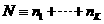. Let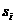,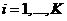, represent the number of treatment response among the ni patients, so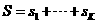. Denote the set of acceptance points (of H0) by (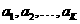) and the set of rejection points (of H0) by (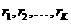). Setting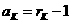General multiple testing procedures (proposed by Schultz et al. (1973)):

At Stage g (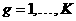),

if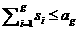, terminate the trial and reject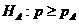;

if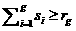, terminate the trial and reject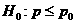;

if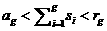, continue to Stage g+1.

(Proposed by O’Brien and Fleming (1979)), at Stage g, would reject H0 whenever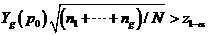where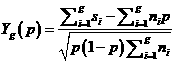Let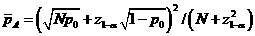, where zp denotes the p-quantile of the standard normal distribution. Rejection of HA at Stage g < K would occur whenever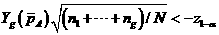.

The corresponding acceptance and rejection points are specified in the following manner: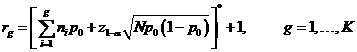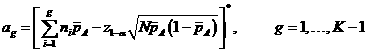where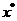denotes the nearest integer to x.

Discussion:

1.    Here is some introduction to single-stage procedure so that readers have a clear mind between the difference of single-stage procedure and multiple testing procedures.

The single-stage procedure can be based upon the statistic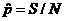, by rejecting H0 whenever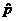is large or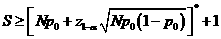.

2.   Fleming has shown that well-designed group sequential procedures are more efficient than single stage procedures in that they require fewer patients to achieve a predetermined size and power.

3.    The multiple testing procedures will require, on the average, fewer patients than the single-stage procedure having similar power. It is more desirable to use a multiple-stage procedure over a single-stage procedure.

4.   A choice of either the Stage 1 or Stage K sample size should not be much less than N/K due to the resulting high degree of conservatism which would be required at a very early first test, and, at Stage K , to a resulting apparent small loss in efficiency.

5.  To obtain useful and efficient designs, approximately equal sample sizes for each stage is recommended.

Statistical Notations:

α:         The probability of type I error (significance level) is the probability of rejecting the true null hypothesis.

β:         The probability of type II error (1 – power of the test) is the probability of not rejecting the false null hypothesis.

Example:

Since Phase I studies indicate that the experimental regimen is somewhat more toxic than the standard, in phase II clinical trial, it is decided to set p0=0.2 and pA=0.4, the maximum 25 patients accrual in 2 stages (Nmax=25, Numstage=2). A one-sided significance level test (α =0.05) with 80% power (β=0.2), the number of patients to accrue in stage 1 is 15 (n1=15) and in the last stage (stage 2), 25 patients are tested (n2=10). The acceptance points and rejection points in stage 1 is 3 and 7 respectively (a1=3, r1=7), it means that if the number of treatment response in stage 1 is less than or equal to 3, then stop the study and reject the alternative hypothesis, if the number of treatment response in stage 1 is greater than or equal to 7, then stop the study and reject the null hypothesis, if the number of treatment response in stage 1 is greater than 3 and less than 7, then continue to stage 2 to recruit 10 more patients. The acceptance points and rejection points in stage 2 is 8 and 9 respectively (a2=8, r2=9), it means that if the number of treatment response in stage 2 is less than or equal to 8, then stop the study and reject the alternative hypothesis, if the number of treatment response in stage 2 is greater than or equal to 9, then stop the study and reject the null hypothesis.

Reference: Fleming (1982) Biometrics 38: 143-151